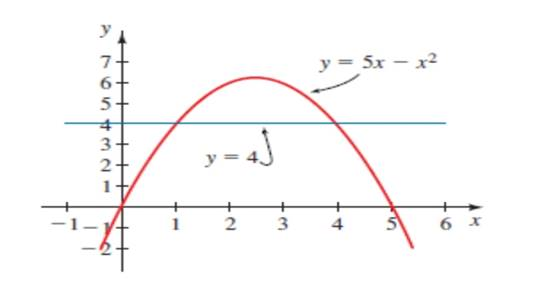# The equation y = 5 x − x 2 and y = 4 ,gives the solution of the equation 5 x − x 2 = 4 .### Precalculus: Mathematics for Calcu...

6th Edition
Stewart + 5 others
Publisher: Cengage Learning
ISBN: 9780840068071### Precalculus: Mathematics for Calcu...

6th Edition
Stewart + 5 others
Publisher: Cengage Learning
ISBN: 9780840068071

#### Solutions

Chapter 1.9, Problem 4E

a.

To determine

## To calculate: The equation y=5x−x2 and y=4 ,gives the solution of the equation 5x−x2=4 .Expert Solution

The solution of the equation y=5xx2 and y=4 is at which the curve touches the xaxis is 1 and 4.

### Explanation of Solution

Given information:

The equation y=5xx2 and y=4 , gives the solution of the equation 5xx2=4 with the help of given graph.

Formula used:

To the help of middle term factorisation method.

Steps to use this method to solve a quadratic polynomial ax2bxc=0 .

Step 1. Multiply the coefficient of x and the constant c .

Step 2. Now to get the coefficient of x , use the resultant value of multiplication of x and c by multiplication or subtraction or division .

Step 3. Now we have 4 terms now, take common from 1st two terms and take common from another two common and from there two factors were come.

Step 3. Take either one bracket is equal to zero or another is equal to zero.

Step 4. Now the value of x comes.

Calculation:

The graph of the equation y=5xx2 and y=4 is sketched:

So find the roots of the equation y=5xx2 and y=4 ,

Rewrite the equation: 5xx24=0

Rearrange the equation in a polynomial form and take ‘-’ common from the equation:

5xx24=0x25x+4=0

Further simplify the equation:

x25x+4=0x24xx+4=0

Now to take common:

x24xx+4=0x(x4)1(x4)=0

Either x1=0 or x4=0 :

Simplify further as: x=1 and x=4

The solution of the equation y=5xx2 and y=4 is at which the curve touches the xaxis is 1 and 4.

b.

To determine

### To calculate: The solution of the equations 5x−x2≻4 .

Expert Solution

The solution of the inequality 5xx24 is x(,1)(4,) .

### Explanation of Solution

Given information:

The inequality 5xx24 .

Formula used:

To the help of middle term factorisation method.

Steps to use this method to solve a quadratic polynomial ax2bxc=0 .

Step 1. Multiply the coefficient of x and the constant c .

Step 2. Now to get the coefficient of x , use the resultant value of multiplication of x and c by multiplication or subtraction or division .

Step 3. Now we have 4 terms now, take common from 1st two terms and take common from another two common and from there two factors were come.

Step 3. Take either one bracket is equal to zero or another is equal to zero.

Step 4. Now the value of x comes.

Calculation:

The inequality 5xx24 .

So find the roots of the inequality 5xx24 ,

Rewrite the inequality: 5xx24=0

Rearrange the equation in a polynomial form and take ‘-’ common from the equation:

5xx24=0x25x+4=0

Further simplify the equation:

x25x+4=0x24xx+4=0

Now to take common:

x24xx+4=0x(x4)1(x4)=0

Either x1=0 or x4=0 :

Simplify further as: x=1 and x=4

Thus, the solution of the inequality 5xx24 is the area of curve above the line y=4

x(,1)(4,) or [1,4] .

### Have a homework question?

Subscribe to bartleby learn! Ask subject matter experts 30 homework questions each month. Plus, you’ll have access to millions of step-by-step textbook answers!